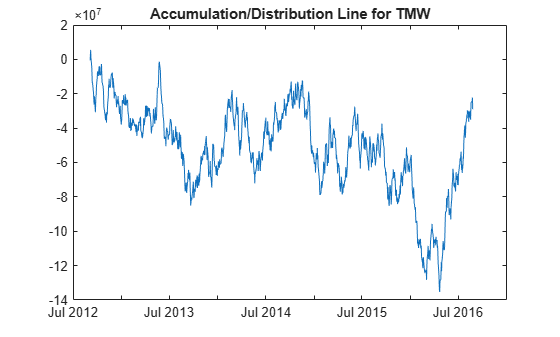Accumulation/Distribution line

`adline` has been partially removed and will no longer accept a `fints` object (`tsobj`) argument. Use a matrix, `timetable`, or `table` instead for financial time series.

Use `fts2timetable` to convert a `fints` object to a `timetable` object.

## Syntax

``ADline = adline(Data)``

## Description

example

````ADline = adline(Data)` calculates the Accumulation/Distribution Line from a set of high, low, closing prices, and volume traded of a security.```

## Examples

collapse all

Load the file `SimulatedStock.mat`, which provides a timetable (`TMW`) for financial data for TMW stock.

```load SimulatedStock.mat line = adline(TMW); plot(line.Time,line.ADLine) title('Accumulation/Distribution Line for TMW')```## Input Arguments

collapse all

Data with high, low, closing prices and volume traded, specified as a matrix, table, or timetable. For matrix input, `Data` is `M`-by-`4` with high, low, closing prices, and volume traded. Timetables and tables with `M` rows must contain a variable named `'High'`, `'Low'`, `'Close'`, and `'Volume'` (case insensitive).

Data Types: `double` | `table` | `timetable`

## Output Arguments

collapse all

Accumulation/Distribution line, returned with the same number of rows (`M`) and the same type (matrix, table, or timetable) as the input `Data`.

 Achelis, S. B. Technical Analysis from A to Z. Second Edition. McGraw-Hill, 1995, pp. 52–53.